The article “Determination of geotechnical characteristic parameters” follows the base principles of the Eurocode.

“The characteristic value of a geotechnical parameter shall be selected as a cautious estimate of the value affecting the occurrence of the limit state.”
[EC7- 2.4.5.2 2(P)]

Defining the geotechnical characteristic value means therefore to choose the geotechnical parameter that influences the behavior of the soil in that particular limit state and adopt a value, or an estimate, in favour of safety.

If statistical methods are used, the characteristic value should be derived such that the calculated probability of a worse value governing the occurrence of the limit state under consideration is not greater than 5%.

Assuming, for exmple, a characteristic value of 26° for the soil friction angle with a 5% fractile, means to hypothesize that there is a probability of five percent that the real value of the friction angle is less that 26°.

A cautios estimate of the geotechnical parameters is obtained through the following formula:where, for a normal distribuiton, for compensation of resistances (slope stability analysis, bearing capacity of shallow foundations)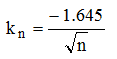in absence of resistance compensation (plinths, bearing capacity of deep foundations)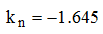In the case of resistances compensation the characteristic value of the geotechnical parameter is close to the mean value.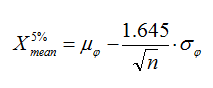In absence of resistances compensation the characteristic value is close to the minimum value.PRACTICAL CASE
It was performed a continous mechanic penetration test to a depth of 6.8 meters from ground level. The test was processed with the software Dynamic Probing, obtaining the values for the angle of shearing resistance shown in the table below: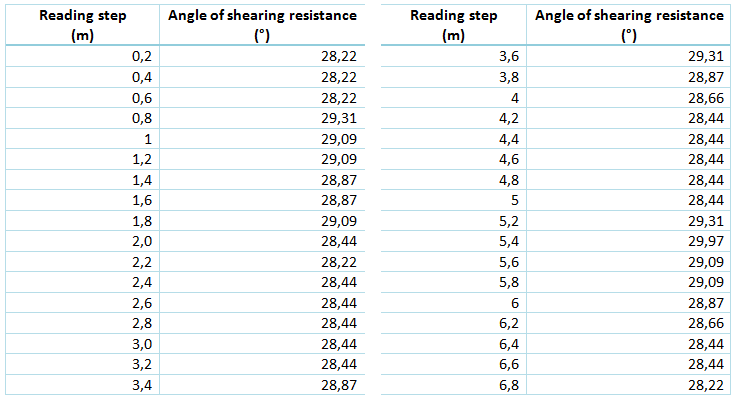According to the indications reported in the literature, the angle of shearing resistance does not follow the normal statistical distribution, but its tangent xi=tan(fi), so we transform the angles in tangent: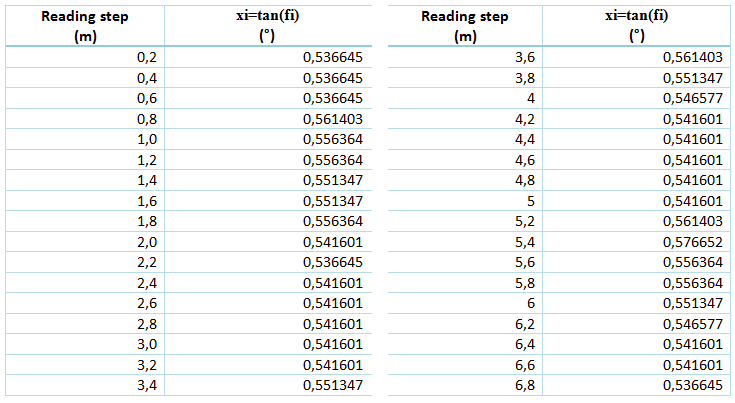Mean value m(xi)= 0,547799;
Standard deviation s(xi)=0,009417

For compensated resistances
The value of the distributed parameter is calculated as:
xi=0,547799-1.645*0,009417/root(34)=0.54518, with the transformation in angle, arctg(0,54518) we obtain 28.6°

For not compensated resistances
The characteristic value of the distributed parameter can be calculated as:
xi=0,547799-1.645*0,009417=0,532307, trasformed in angle arctg(0,532307)=28.0°

In this case (large sample) the difference between the obtained values is minimal, but the difference may become substantial for small samples (n<30) as in the majority of cases and for some geotechnical parameters, such as cohesion, which distributes its statistical value according to a logarithmic law.

The software CVSOIL offers the possibility to analyze: numerous samples, dispersed samples, highly dispersed saples, linear distribution, tangent, logarithmic etc.
CVSOIL simplifies the user’s work allowing to automatically acquire the data to estimate from Excel, memory or directly from the software Static Probing and  Dynamic Probing.

In this short video you can see how data is beeing processed in CVSOIL

The estimation of the characteristic value of the geotechnical parameters is very variable especially when the samples are highly dispersed. CVSOIL has the statistical models that follow the principles of the Eurocodes and which allow to make an accurate estimate of the characteristic value of the analyzed parameter even in the case of very dispersed samples.